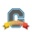cancel
Showing results for
Did you mean:
Highlighted
Surveyor

Hello. How can I create a custom grading scheme with fixed point values instead of ranges?

For example: A=95, B=85, instead of A=100-90 and B=89-80

2 Replies
HighlightedCommunity Coach

As far as I know, even if you set your total column in the gradebook to display as points, all grading schemes in Canvas are based on percentages of total points possible. So what you are asking to do, is really not possible without hinking the system a bit, and even then it will not be exactly what you are looking for.

For example, if you want 95 points to equal an A (what happens to students who get 96 points?), then determine what percentage of the total possible points 95 would be (we will just assume 95 for this example), then you would set the range to be from 95.00 to 95.01.

All of this begs the question already asked, what happens to students who get 96 points, or 86 points? Do they just not get a letter grade, or do you not want a letter grade displayed for them?

Kelley

Tags (1)
Highlighted
Surveyor

Since my course is an English composition course, I give fixed letter grades (eg. A, B, C, etc). It is not like a math test where a student can earn a range of points that are not fixed. Thus, no student in my course will earn an 86, 96, etc. However, Canvas does not have the option to put in fixed grades, instead taking an average in a letter grading system. For example, if I set the range of A to be 90-100, and I give the grade A, Canvas seems to automatically award a 95. This does not work for me, as I only award fixed letter grades.

Now, if I set a range from 95.00-95.01, the next range, which I would like to be 90, automatically starts at 95 now as it is continuous. There is no option to set non-continuous ranges.

Is there any way to request a grading scheme that allow fixed percentages assigned to letter grades?